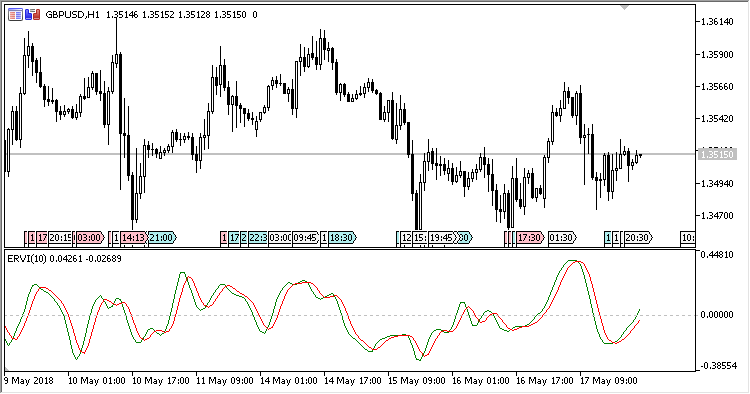Interesting script?
So post a link to it -
let others appraise it

You liked the script? Try it in the MetaTrader 5 terminal# ERVI - indicator for MetaTrader 5

Views:
553
Rating:
Published:
2018.06.18 10:26
Updated:
2018.10.04 10:25

Oscillator ERVI (Ehlers' Relative Vigor Index) based on the relative vigor index by John F. Ehlers.

It has one input parameter:

• Period - calculation period.

Calculations:

```RVI[i] = Num/Denom
Signal[i] = (RVI[i] + 2*RVI[i-1] + 2*RVI[i-2] + RVI[i-3])/6
```

where:

```Num = SMA(Value1, Period)
Denom = SMA(Value2, Period)
Value1[i] = ((Close[i] - Open[i]) + 2*(Close[i-1] - Open[i-1]) + 2*(Close[i-2] - Open[i-2]) + (Close[i-3] - Open[i-3]))/6
Value2[i] = ((High[i] - Low[i]) + 2*(High[i-1] - Low[i-1]) + 2*(High[i-2] - Low[i-2]) + (High[i-3] - Low[i-3]))/6
```Translated from Russian by MetaQuotes Software Corp.
Original code: https://www.mql5.com/ru/code/20798ESM

Indicator ESM (Elsig's Shifting Mean) shows the averaged shift of price over the specified time interval (bars).Advance_Decline_Line

Indicator ADL (Advances/Declines Line) shows the ratio of positive and negative price increments over a specified time interval.GAPO

GAPO (Gopalakrishnan Range Index Oscillator) assesses quantitatively the volatility of an instrument, based on the trading range logarithm over an N-day period of time.Glitch_Index

Glitch_Index - indicator of the price deviation from its average value.